We’ll be covering while loop in this tutorial.

A while loop let you do repeated execution of one or more lines of code, until the boolean condition changes.

The code block inside the while loop (four spaces indention) will execute as long as the boolean condition in the while loop is True.

Related Course: Complete Python Programming Course & Exercises

## While Loop syntax

While loops exist in many programming languages, it repeats code.

Python’s while loop has this syntax:

`<statements>` are one or more lines of code, they must be indented with four spaces.

The statements repeat until the expression changes.

The expression `<exp>` is evaluated in Boolean context.

• If the expression `<expr>` is True, the loops body is executed.

• While it stays True, it keeps repeating.

• If the expression `<expr>` becomes False, it ends the loop.

## Python while loop

Lets build a program that has a `while` loop:

This is what happens:

• the expression `<expr>` is `i < 6`, the code below repeats while True.

• it has two statements.

• 1st statement `print(f"i = {i}")` outputs variable `i`.

• 2nd statement `i = i + 1` increases i.

• the while loop stops when the `<expr>` is False: `i >= 6`.

Lets have a look: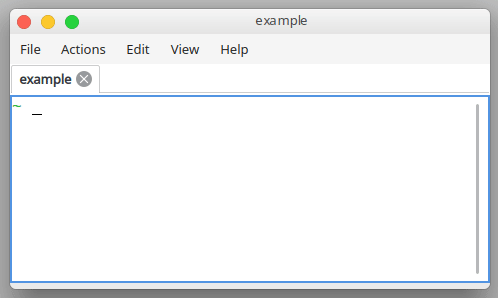The program outputs:

So why is 6 not included?

Because if a is equal to 6, the condition `i < 6` is False.

To include six, change it to `<=`.

You can make a countdown timer with a while loop:

This will repeat until variable i is equal to one.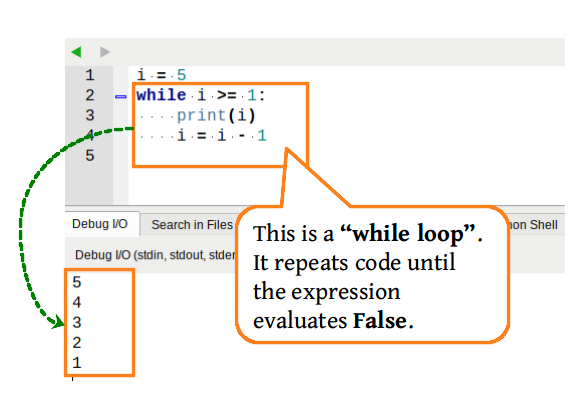Related Course: Complete Python Programming Course & Exercises

## While loop flow chart

The flow chart below shows the while loop:If the boolean condition of a `while` loop is never changes, it could cause the program to run forever or to freeze/crash.

To make an infinite while loop, run the code below:

A while loop terminates if the expression is False.

You can end execution by pressing `Ctrl-Z` in the Python shell.

## Break and continue

The statements break and continue modify the loops behavior.

• the break statement exits the loop.

• the continue statement skips the current cycle.

The program below uses the break statement:

break terminates the loop.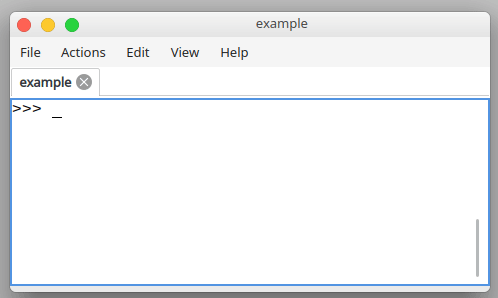The example below shows the continue statement:

It skipped the third iteration because continue was used.

The continue statement skips one or more cycles.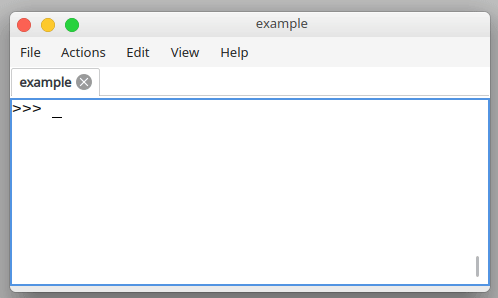## The else clause

The else clause can be used with a while loop.

This is unique to Python.

The syntax is:

The `<other_statement(s)` will only be executed in normal execution flow.

If the while loop ends because of the break keyword, it won’t be run.

The eample below does normal execution:

If break is called before ending the while loop, the else clause isn’t run.

That’s because the while loop is terminated prematurely with break.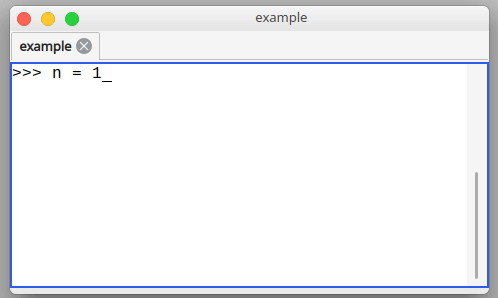Related Course: Complete Python Programming Course & Exercises

## One-Line while loops

While loop can be one a single line, where multiple statements are separated by a semicolon `;`.

This behaves exactly as the multiline loop, it outputs:

But this only works for very simple while loops. You can’t add if statements inside.

PEP 8 discourages multiple statements on a single line.

## Python while loop input

You can get a number from the keyboard using this line:

It gets keyboard input with the input() function that returns text.

That text is then convert to a number with `int()`.

The above expression `x != 5` will repeat until x is equal to five.

Then it keeps asking the user to input the number and only quits the loop when x is equal `==` to five.

This will keep asking a number until the number is guessed.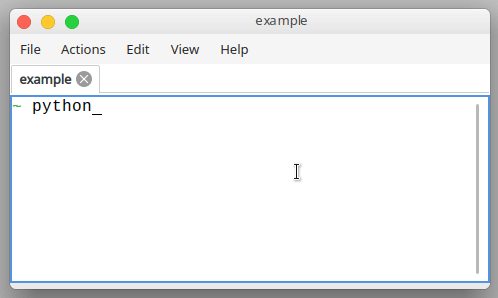This is defined in the line: `while x != 5`, or in English, “while x is not equal to five, repeat”.

The program keeps repeating the code block while the condition `(x != 5)` is True.

If x is equal to five, the program ends the loop.

## Conclusion

In this tutorial, you learned about while loops.

• a while loop is indefinite iteration
• a while loop repeats code while the expression is True
• it exits the loop if the expression is False
• the break and continue statements change the loop behavior

If you are a Python beginner, then I highly recommend this book.